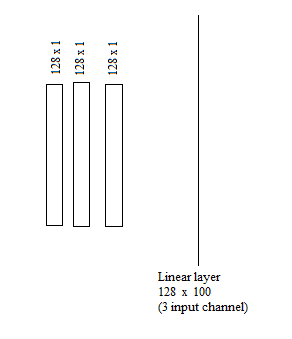# Create FC layer with multiple input channel and one output channel

Hi,
I have 3 feature vector with 128 x 1 dimensions, in the last classification layer, I would like to feed each feature vector as one channel to final FC layer but as you know Pytorch does not support linear layer with multiple input channel.What I want is a linear layer with:
Input: 3 channel: each channel 128x1 feature vector
output: 1 channel 100 class probabilities.

Is it different than concatenating all 3 feature vectors and feed to linear layer?
384*1 single channel feature vactor?

Could you describe what “channels” would represent in a linear layer?
`nn.Linear` accepts inputs with an additional dimension as `[batch_size, *, in_features]` and will be applied sequentially on the additional dims, but I don’t understand what a channel would represent here.

Each channel is the output of one specific feature extractor. For example assume we have 3 different encoders as feature extractors (F1,F2,F3). Each encoder have different loss function or depth or architecture.
In the final classification layer we want to use all information extracted in each encoders. One simple method is feeding the output of all of those pre-trained encoders as channels to the final classification linear layer. In the linear evaluation protocol we cannot add more than single linear layer so I want to add just one linear layer with multiple input channel.
1-I can concatenate all encoders output and fed to one channel linear layer but I 'm not sure what will happened in back-propagation? Could it distinguish between each encoder feature space? Could it learn properly in comparison to multi-channel input?
2-After that I can use channel attention to learn suitable view and use the output of attention as the input of linear layer.

The issue is that a linear layer doesn’t have any notion of “channels” and expects “features” while your explanation points to feed the input “as channels” to the linear layer.

If you want to sequentially apply the linear layer to each of these “channels” (given as an additional dimension in your output) you could directly pass them to the layer as:

``````x = torch.randn(2, 3, 4)
lin = nn.Linear(in_features=4, out_features=7)

out = lin(x)
print(out.shape)
# torch.Size([2, 3, 7])
``````

Here the linear layer will be applied on each index in `dim1` separately as if you would iterate this dimension.

Again, the same issue that linear layers don’t work on channels but pure features. Concatenating tensors will not break the computation graph and you will be able to calculate the gradients. I don’t know how you want to apply the linear layer on the inputs so also cannot comment on its ability to learn properly.

1 Like

Thanks, I saw similar answer to previous question here. I present my implementation for concatenating the encoders output and feed them as the input of linear layer.

``````class Res50_test(nn.Module):
def __init__(self, st):
super().__init__()
self.encoders=nn.ModuleList()

for i in range(3):
self.encoders.append(torchvision.models.resnet50(zero_init_residual=True))
for child in self.encoders.children():
for param in child.parameters():

sizes = [2048*3,100]
self.classifier = nn.Linear(sizes[-2], sizes[-1], bias=False)

def forward(self, x):
emb=[]
for i,encoder in enumerate(self.encoders):
emb.append(encoder(x))
emb=torch.cat(emb,dim=1)
logit=self.classifier(emb)
return logit,emb
``````

I wanted to differentiate between the output effect of each encoder in classifier.

Thanks for the link. The author of this post shared this:

``````Here is the pseudo code:

input_matrix[ X x Y x Z x Channels]

linear_layer = nn.linear( in_channel, out_channel)

output_matrix = linear_layer(input_matrix)
``````

which fits my code snippet and will apply the linear layer on the additional dimensions.

Your approach also seems to use the same approach by creating the additional `dim1` in `emb`.

1 Like

Yes, You are right, your answer for that(link) question is correct. But if I use additional dim1 in emb and feed it to the linear layer, the output shape will be (3,100). It means that the classifier considers each feature space (encoder) separately and creates 3 logits for each class. I want the to consider them together but differentiate between each encoders output.
I think, as you said before, there are not any notion of “channels” for linear layers so my only remaining solution is concatenating them. Do you have any idea to differentiate between each encoders output as the input of linear layer?

In that case I might have misunderstood your code snippet and it seems `emb` is still 2-dimensional.
Try to follow the approach form my code snippet and `stack` the features in a new dimension at `dim1`.

1 Like